# Convergence2

Convergence for Eigenvalue Problems with Mesh Adaptivity

A posteriori analysis:

This section contains our a posteriori error estimator and the definition of the mesh adaptivity algorithm for which convergence will be proved in the following sections.

Recalling the mesh sequencedefined above, we letdenote the set of all the edges (orthe set of faces in 3D) of the elements of the mesh. For each, we assume that we have already chosen a preorientated unit normal vectorand we denote byandthe elements sharing(i.e.). In addition we write. Elements, facesnand edges are to be considered closed. Furthermore we denote the diameter ofby.

Notation 2.1 We writewhen A/B is bounded by a constant which may depend on the functionsandin (1.2), on,,and, onin Assumption 1.1 and onin (1.7). The notationmeansand.

All the constants depending on the spectrum are handled explicitly. Similarly all mesh size dependencies are explicit. Note that all eigenvalues of (1.6) satisfy, since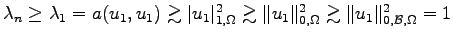.

The error estimator which we shall use is obtained by adapting the standard estimates for source problems to the eigenvalue problem.

For a function, which is piecewise continuous on the mesh, we introduce its jump across an edge (face)by:

Then for any function  with piecewise continuous gradient onwe define, forforThe error estimator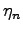on the meshis defined as

where each term, which is the local contribution to the residual, is defined by

The following lemma is proved, in a standard way, by adapting the usual arguments for source problems.

Lemma 2.1 (Reliability)

and

Remark 2.2 We shall see below that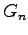defined above constitutes a “higher order term”.

The idea is to refine a subset of the elements ofwhose side residuals sum up to a fixed proportion of the total residual.

Definition 2.4 (Marking Strategy 1) Given a parameter, the procedure is: mark the sides in a minimal subsetofsuch that

To satisfy the condition (2.5), we need first of all to compute all the “local residuals”and sort them according their values. Then the edges (faces)are inserted intoin decreasing order of, starting from the edge (face) with the biggest local residual, until the condition (2.5) is satisfied. Note that a minimal subsetmay not be unique. Then we construct another set, containing all the elements ofwhich share at least one edge (face).

In order to prove the convergence of the adaptive method, we require an additional marking strategy, which will be defined in Definition 2.6 below. The latter marking strategy is driven by oscillations. The same argument has been already used in many papers about convergence for source problems, but to our knowledge has not yet been used for analysing convergent algorithms for eigenvalue problems.

The concept of “oscillation” is just a measure of how well a function may be approximated by piecewise constants on a particular mesh. For any function, and any mesh, we introduce its orthogonal projectiononto piecewise constants defined by: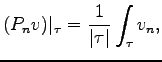for all(2.6)

Then we make the definition:

Definition 2.5 (Oscillations) On a mesh, we define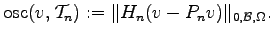(2.7)

Note that

and that (by standard approximation theory and the ellipticity of,for all(2.8)

The second marking strategy (introduced below) aims to reduce the oscillations corresponding to a particular approximate eigenfunction.

Definition 2.6 (Marking Strategy 2) Given a parameter: mark the sides in a minimal subsetofsuch that Note that a minimal subsetmay not be unique. To satisfy the condition (2.9), we need first of all to compute all the local termsformingand sort them according their values. Then the elementsare inserted intoin decreasing order of the size of those local terms, until the condition (2.9) is satisfied.

Our adaptive algorithm can then be stated:In 2D at the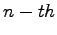iteration in Algorithm 1 each element in the setis refined using the “bisection5” algorithm, as illustrated in Figure 1c. An advantage of this technique is the creation of a new node in the middle of each marked side inand also a new node in the interior of each marked element.Figure 1: The refinement procedure applied to an element of the mesh. In (a) the element before the refinement, in (b) after the three sides as been refined and in (c) after the bisection of one of the three new segments.